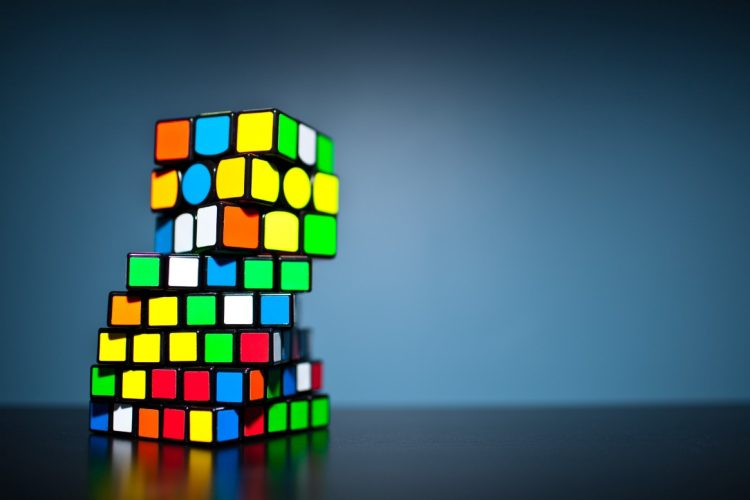# Find Patterns, Solve Problems

By Math Is Fun, August 14, 2022

“What’s the last digit of 18100?” I asked my son all of a sudden.

“Wow, it will take some time to calculate the answer!” Son replied.

“I can tell you the answer in a few seconds. So can you.”

“Really?”

“Yes, try it and I believe you can find the answer.”

“OK! Let’s see. What’s the last digit of 18 * 18? 8 multiples 8 is 64, so it’s 4.”

“Great! To solve a complex problem, start with a small step.” I summarized. “So what observation did you have with this simpler case?”

“Ah, since we only need the last digit of 18100, we do not care about any other digits. So we only need to know the last digit of 8100.”

“Cool, please continue.” I encouraged him.

“82 ends in 4. 4 multiples 4 is 16. So 84 ends in 6. Ah! 6 is an automorphic number! Since 6 multiples 6 always ends in 6, and 100 is a multiple of 4, the last digit of 8100 is 6!” Son is pretty excited to find the answer. (For our readers’ reference, we previously discussed automorphic numbers in this episode.)

“Ha! Did I tell you you can solve this problem quickly?” I said with a smile. “When there is a problem that seems difficult, you can try to find patterns in the problem, and sometimes you will be surprised how easy the solution is!

Now, let’s extend the problem further. What’s the last digit of 18101?“

“Since the last digit of 18100 is 6. 6 multiplies 8 is 8. The last digit of 18101 is 8. Similarly the last digit for 18102 is 4. The last digit for 18103 is 2. Then we are back to 6 as the last digit for 18104.”

“Cool! For 18 to the power of any number, we can tell the last digit very quickly.” I continued to expand the question. “What about the powers of any other numbers? Can we tell the last digit?”

“Let’s see. For numbers ending in 0, 1, 5, 6, they will keep the last digits since these are automorphic numbers. For 2 as the end digit, 2 multiples 2 is 4, 4 multiples 4 is 16. Here we go! 2 is similar to 8.”

It turns out, numbers ending with any digit have similar patterns. In all cases, the last digit will repeat itself every 4th power (d4, d8, d12,… have the same last digit). So it shouldn’t take more than a few seconds to answer the question: what’s the last digit of a to the power of b.

The important lesson of this episode: To solve a complex problem (whether it’s a math problem, or a real life problem)